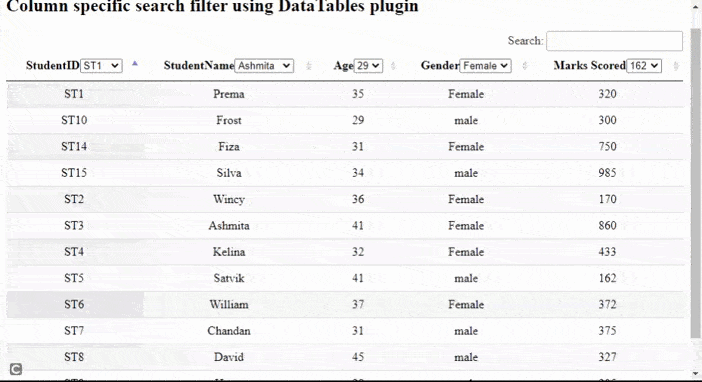# How to implement column specific search filter using DataTables plugin ?

• Difficulty Level : Medium
• Last Updated : 11 Nov, 2021

DataTables are a modern jQuery plugin for adding interactive and advanced controls to HTML tables for a webpage. It is a very simple-to-use plug-in with a variety of options for the developer’s custom changes as per the application’s need. The plugin’s features include pagination, sorting, searching, and multiple-column ordering.

In this article, we will demonstrate the implementation of a column-specific search filter using the DataTables plugin. Instead of performing search operations on the whole table, the search is performed only on a particular column for improving the performance of the system

Attention reader! Don’t stop learning now. Get hold of all the important HTML concepts with the Web Design for Beginners | HTML  course.

Approach: In the following example, DataTables uses the student details from the HTML table as the main source. Each row in the table shows details for one student’s information.

• A DataTable is initialized.
• The developer can set the features of paging or searching as per the need as shown in the script part of the code.
• The column() API is used to select all the columns of the table.
• The flatten() API is used to convert the 2D array structure into a single dimension array and each() method is used to perform any action on each of the selected columns.
• The select list is appended to each column header.
• Any action is performed on change of any list value.
• The column().search() API is used to search the selected value and the draw() API is used to reflect the changes after the action in the table.
• column().cache() API is used to get the data from the column with sort() method to display sorted data.
• All the steps are done for each of the columns.

The pre-compiled files which are needed to implement are

CSS CDN:

`https://cdn.datatables.net/1.10.22/css/jquery.dataTables.min.css`

JavaScript CDN:

```https://code.jquery.com/jquery-3.5.1.js
https://cdn.datatables.net/1.10.22/js/jquery.dataTables.min.js```

Example:  The following example demonstrates the above approach showing column-specific search filter operation.

## HTML

 ```<``html``>` `<``head``>``  ``<``meta` `content="``initial-scale``=``1``,``         ``maximum-scale``=``1``, ``user-scalable``=``0``" ``name``=``"viewport"` `/>``  ``<``meta` `name``=``"viewport"` `content``=``"width=device-width"` `/>` `  ````  ``<``link` `rel``=``"stylesheet"` `href``=``"https://cdn.datatables.net/1.10.22/css/jquery.dataTables.min.css"` `/>``  ````  ``<``script` `type``=``"text/javascript"` `src``=``    ``"https://code.jquery.com/jquery-3.5.1.js"``>``  ````  ` `  ````  ``<``script` `type``=``"text/javascript"` `src``=``"https://cdn.datatables.net/1.10.22/js/jquery.dataTables.min.js"``>``  ``` `  ``<``style``>``    ``td {``      ``text-align: center;``    ``}``  ````` `<``body``>``  ``<``h2``>``    ``Column specific search filter``    ``using DataTables plugin``  ``` `  ````  ``<``table` `id``=``"tableID"` `class``=``"display"``      ``style``=``"width: 100%"``>``    ``<``thead``>``      ``<``tr``>``        ``<``th``>StudentID``        ``<``th``>StudentName``        ``<``th``>Age``        ``<``th``>Gender``        ``<``th``>Marks Scored``      ````    ````    ``<``tbody``>``      ``<``tr``>``        ``<``td``>ST1``        ``<``td``>Prema``        ``<``td``>35``        ``<``td``>Female``        ``<``td``>320``      ````      ``<``tr``>``        ``<``td``>ST2``        ``<``td``>Wincy``        ``<``td``>36``        ``<``td``>Female``        ``<``td``>170``      ````      ``<``tr``>``        ``<``td``>ST3``        ``<``td``>Ashmita` `        ``<``td``>41``        ``<``td``>Female``        ``<``td``>860``      ````      ``<``tr``>``        ``<``td``>ST4``        ``<``td``>Kelina``        ``<``td``>32``        ``<``td``>Female``        ``<``td``>433``      ````      ``<``tr``>``        ``<``td``>ST5``        ``<``td``>Satvik``        ``<``td``>41``        ``<``td``>male``        ``<``td``>162``      ````      ``<``tr``>``        ``<``td``>ST6``        ``<``td``>William``        ``<``td``>37``        ``<``td``>Female``        ``<``td``>372``      ````      ``<``tr``>``        ``<``td``>ST7``        ``<``td``>Chandan``        ``<``td``>31``        ``<``td``>male``        ``<``td``>375``      ````      ``<``tr``>``        ``<``td``>ST8``        ``<``td``>David``        ``<``td``>45``        ``<``td``>male``        ``<``td``>327``      ````      ``<``tr``>``        ``<``td``>ST9``        ``<``td``>Harry``        ``<``td``>29``        ``<``td``>male``        ``<``td``>205``      ````      ``<``tr``>``        ``<``td``>ST10``        ``<``td``>Frost``        ``<``td``>29``        ``<``td``>male``        ``<``td``>300``      ``` `      ``<``tr``>``        ``<``td``>ST14``        ``<``td``>Fiza``        ``<``td``>31``        ``<``td``>Female``        ``<``td``>750``      ````      ``<``tr``>``        ``<``td``>ST15``        ``<``td``>Silva``        ``<``td``>34``        ``<``td``>male``        ``<``td``>985``      ````    ````  ````  ``<``br` `/>` `  ``<``script``>` `      ``/* Initialization of datatables */``      ``\$(document).ready(function () {` `        ``// Paging and other information are``        ``// disabled if required, set to true``        ``var myTable = \$("#tableID").DataTable({``          ``paging: false,``          ``searching: true,``          ``info: false,``        ``});` `        ``// 2d array is converted to 1D array``        ``// structure the actions are``        ``// implemented on EACH column``        ``myTable``          ``.columns()``          ``.flatten()``          ``.each(function (colID) {` `            ``// Create the select list in the``            ``// header column header``            ``// On change of the list values,``            ``// perform search operation``            ``var mySelectList = \$("<``select` `/>")``              ``.appendTo(myTable.column(colID).header())``              ``.on("change", function () {``                ``myTable.column(colID).search(\$(this).val());` `                ``// update the changes using draw() method``                ``myTable.column(colID).draw();``              ``});` `            ``// Get the search cached data for the``            ``// first column add to the select list``            ``// using append() method``            ``// sort the data to display to user``            ``// all steps are done for EACH column``            ``myTable``              ``.column(colID)``              ``.cache("search")``              ``.sort()``              ``.each(function (param) {``                ``mySelectList.append(``                  ``\$('<``option` `value``=``"' + param + '"``>'``                    ``+ param + "")``                ``);``              ``});``          ``});``      ``});``  ````` ``

Output:My Personal Notes arrow_drop_up